# Inverse Trigonometric functions Questions and Answers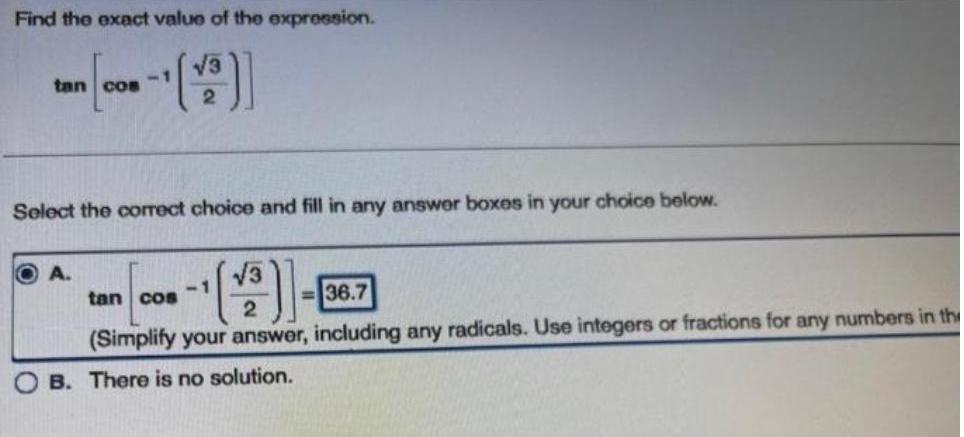Math
Inverse Trigonometric functions
Find the exact value of the expression. tan cos[-1(√3/2)] Select the correct choice and fill in any answer boxes in your choice below. A.=tan cos[-1(√3/2)]=36.7 (Simplify your answer, including any radicals. Use integers or fractions for any numbers in the B. There is no solution.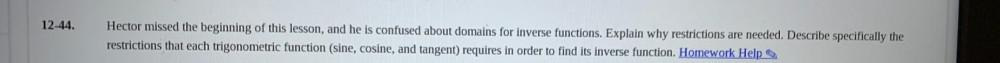Math
Inverse Trigonometric functions
Hector missed the beginning of this lesson, and he is confused about domains for inverse functions. Explain why restrictions are needed. Describe specifically the restrictions that each trigonometric function (sine, cosine, and tangent) requires in order to find its inverse function.Math
Inverse Trigonometric functions
Find the value of the following: cos(tan-¹ 4/9)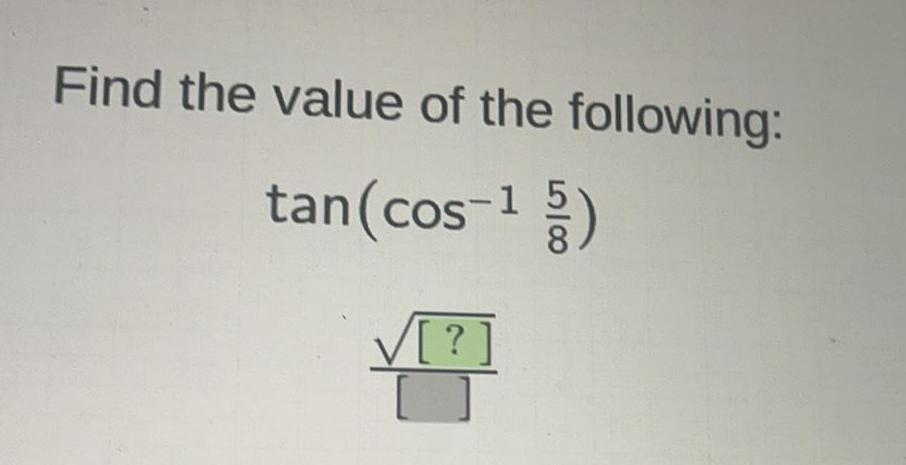Math
Inverse Trigonometric functions
Find the value of the following: tan(cos-¹ 5/8) √(?)/ ( )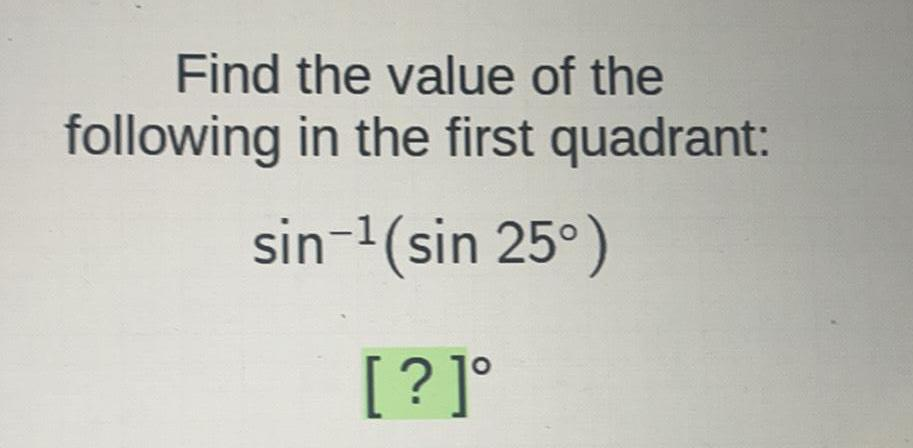Math
Inverse Trigonometric functions
Find the value of the following in the first quadrant: sin-¹ (sin 25°) [?]°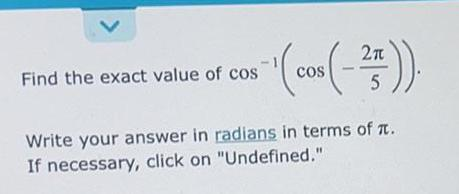Math
Inverse Trigonometric functions
Find the exact value of cos^-1(cos(-2π/5)). Write your answer in radians in terms of π. If necessary, click on "Undefined."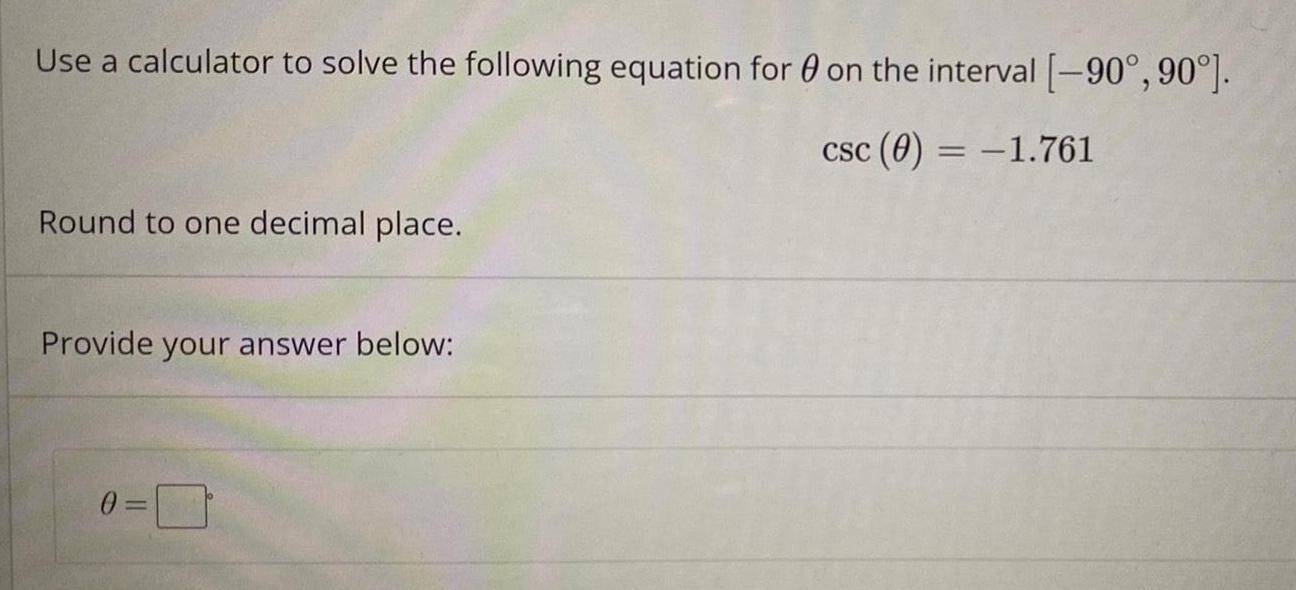Math
Inverse Trigonometric functions
Use a calculator to solve the following equation for on the interval [-90°, 90°]. csc (θ) = -1.761 Round to one decimal place. Provide your answer below: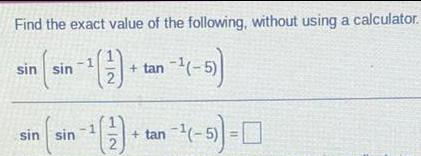Math
Inverse Trigonometric functions
Find the exact value of the following, without using a calculator. sin (sin-¹(2) + tan -1(-5) sin sin -¹+tan ¹(-5)- -0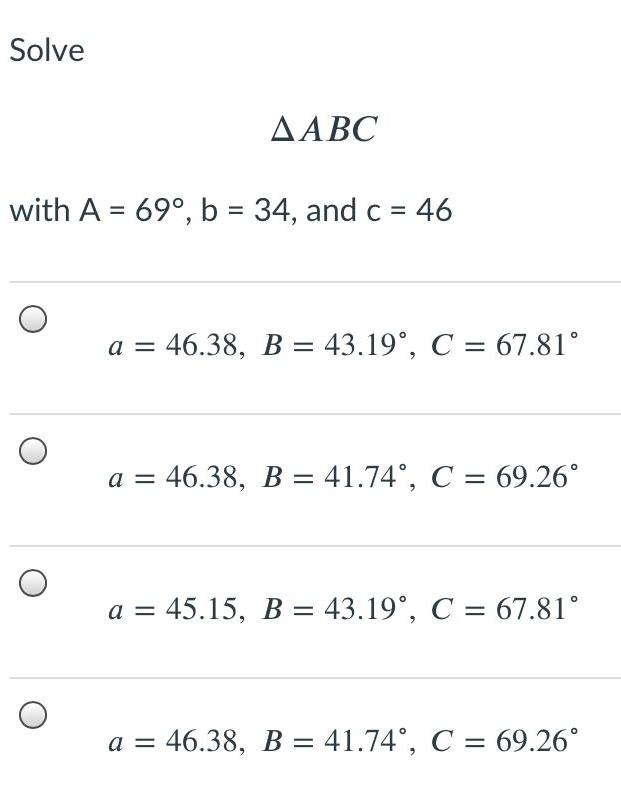Math
Inverse Trigonometric functions
Solve ΔABC with A = 69°, b = 34, and c = 46 a = 46.38, B = 43.19°, C = 67.81° a = 46.38, B = 41.74°, C = 69.26° a = 45.15, B = 43.19°, C = 67.81° a = 46.38, B = 41.74°, C = 69.26°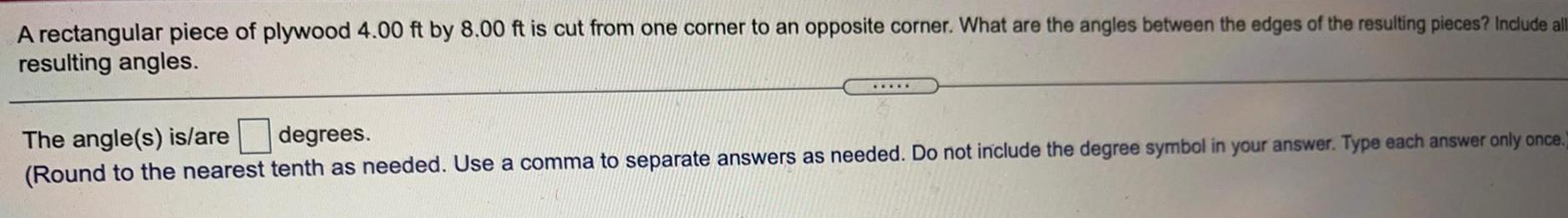Math
Inverse Trigonometric functions
A rectangular piece of plywood 4.00 ft by 8.00 ft is cut from one corner to an opposite corner. What are the angles between the edges of the resulting pieces? Include all resulting angles.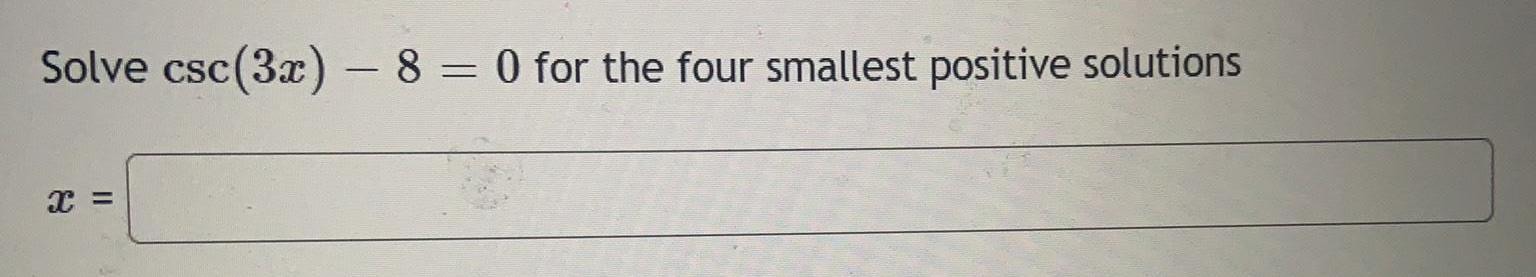Math
Inverse Trigonometric functions
Solve csc (3x) - 8 = 0 for the four smallest positive solutions x =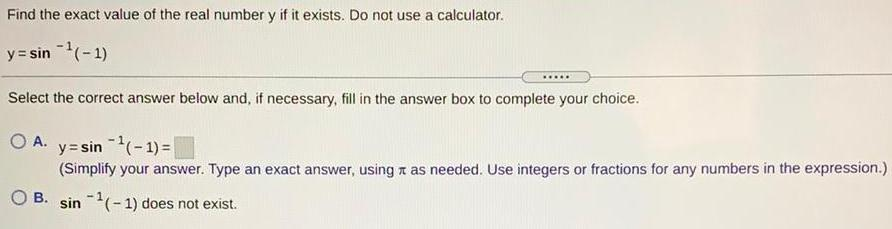Math
Inverse Trigonometric functions
Find the exact value of the real number y if it exists. Do not use a calculator. y=sin ^-1¹(-1) Select the correct answer below and, if necessary, fill in the answer box to complete your choice. A. y=sin ¹(-1) = (Simplify your answer. Type an exact answer, using as needed. Use integers or fractions for any numbers in the expression.) B. sin 1(-1) does not exist.
1